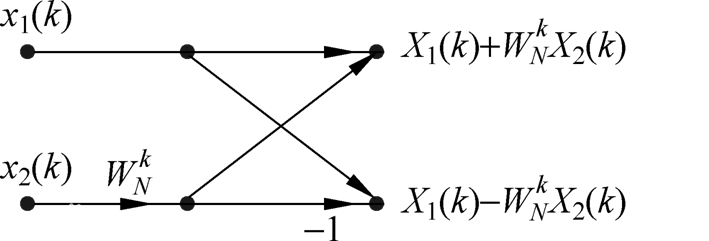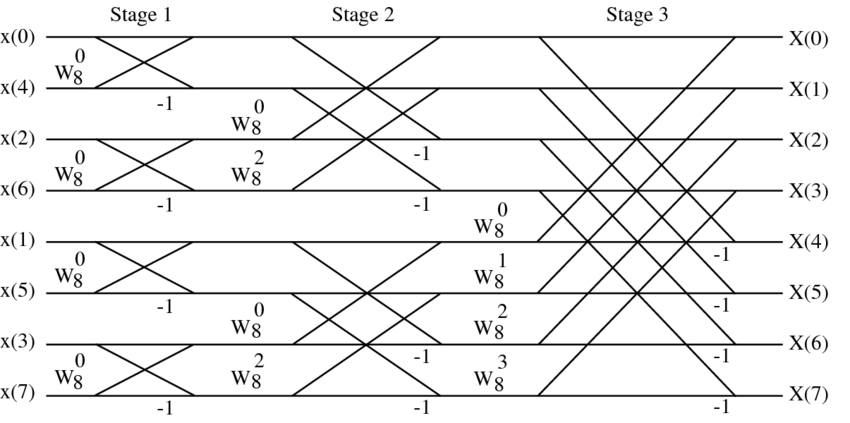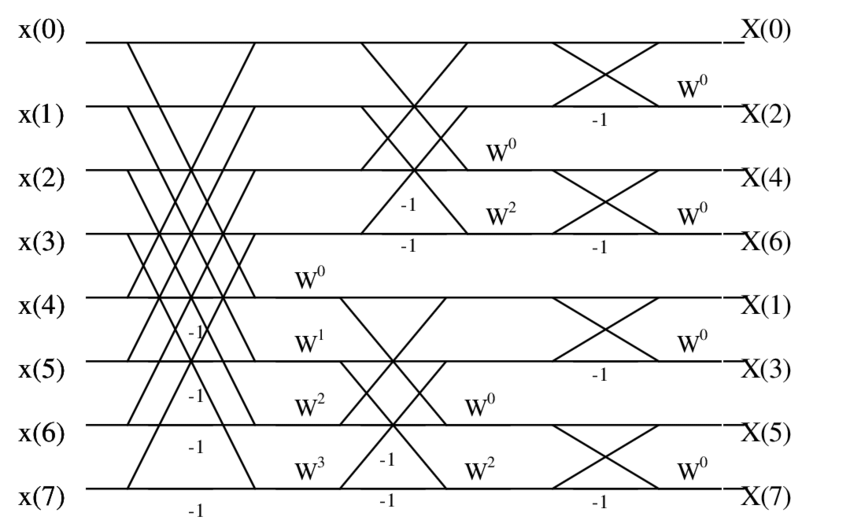# FFT

\begin{align*} \newcommand{\dif}{\mathop{}\!\mathrm{d}} \newcommand{\belowarrow}{\mathop{#1}\limits_{\uparrow}} \newcommand{\bd}{\boldsymbol} \newcommand{\L}{\mathscr{L}} \newcommand{\red}{\color{red}} \newcommand{\xleftrightarrow}{\stackrel{#1}{\longleftrightarrow}} \end{align*}

$X[k]=\sum_{n=0}^{N-1}x[n]W_N^{kn},\;k=0,1,\cdots,N-1\\ W_N^{kn}=e^{-2\pi kn/N}$

# 基-2 FFT

## DIT-FFT 算法

\begin{aligned} x_1 [r] &= x[2r]\\ x_2 [r] &= x[2r+1] \end{aligned}\; r=0,1,\cdots,\frac{N}{2}-1

\begin{aligned} X[k]&=\sum_{n = 偶} x[n] W_N^{kn}+\sum_{n = 奇} x[n] W_N^{kn}\\ &=\sum_{r=0}^{N/2-1} x[2r] W_N^{2kr}+W_N^{k} \sum_{r=0}^{N/2-1} x[2r+1] W_N^{2kr}\\ &=\sum_{r=0}^{N/2-1} x[2r] (W_N^{2})^{kr}+W_N^{k} \sum_{r=0}^{N/2-1} x[2r+1] (W_N^{2})^{kr} \end{aligned}

\begin{aligned} X[k]&=\sum_{r=0}^{N/2-1} x[2r] W_{N/2}^{kr}+W_N^{k} \sum_{r=0}^{N/2-1} x[2r+1] W_{N/2}^{kr}\\ &=X_1[k]+W_N^k X_2[k] \quad k=0,1,\cdots,N-1 \end{aligned}

$X[k+\frac{N}{2}] = X_1[k]-W_N^k X_2[k] \quad k=0,1,\cdots,N-1$$\log_2 N \begin{cases} \quad\quad\quad\quad N\\ \quad\quad\quad\swarrow \quad\searrow\\ \quad\quad N/2 \quad\; N/2\\ \quad\;\swarrow \quad\searrow \;\; \swarrow \quad\searrow\\ N/4 \;\; N/4 \; N/4 \;\; N/4\\ \quad\quad\quad\quad\;\vdots\\ \end{cases}$$X_0=00\red0$ $X_1=00\red1$ $X_2=01\red0$ $X_3=01\red1$ $X_4=10\red0$ $X_5=10\red1$ $X_6=11\red0$ $X_7=11\red1$ $00\red0$$01\red0$$10\red0$$11\red0 00\red1$$01\red1$$10\red1$$11\red1$ $0\red00$$1\red00 0\red10$$1\red10$ $0\red01$$1\red01 0\red11$$1\red11$ $x_0=\red000$ $x_4=\red100$ $x_2=\red010$ $x_6=\red110$ $x_1=\red001$ $x_5=\red101$ $x_3=\red011$ $x_7=\red111$

$\Psi_{r+1}[\alpha] = \Psi_r[\alpha]+W_N^\ell \Psi_r[\beta]\\ \Psi_{r+1}[\beta] = \Psi_r[\alpha]-W_N^\ell \Psi_r[\beta]$

$\alpha$，$\beta$，$r$，$\ell$ 之间满足一些关系：

1. $\alpha=a\cdot 2^r+b$，其中，$a=0,1,\cdots,N/2^r-1$，而 $b=0,1,\cdots,2^{r-1}-1$
2. $\beta$ 与 $\alpha$ 一一对应，$\beta=\alpha+2^{r-1}$
3. $\ell=b\cdot N/2^r$

## DIF-FFT

\begin{aligned} X[2r]&=\sum_{n=0}^{N-1} x[n] W_N^{n(2r)}\\ &=\sum_{n=0}^{N/2-1} x[n] W_N^{n(2r)}+\sum_{n=N/2}^{N-1} x[n] W_N^{n(2r)}\\ &=\sum_{n=0}^{N/2-1} x[n] W_N^{n(2r)}+W_N^{Nr}\sum_{n=0}^{N/2-1} x[n+N/2] W_N^{n(2r)}\\ &=\sum_{n=0}^{N/2-1} (x[n]+x[n+N/2]) W_{N/2}^{nr}\,,r=0,1,\cdots,N/2-1 \end{aligned} \begin{aligned} X[2r+1]&=\sum_{n=0}^{N-1} x[n] W_N^{n(2r+1)}\\ &=\sum_{n=0}^{N/2-1} x[n] W_N^{n(2r+1)}+\sum_{n=N/2}^{N-1} x[n] W_N^{n(2r+1)}\\ &=\sum_{n=0}^{N/2-1} x[n] W_N^{n(2r+1)}+W_N^{(Nr+N/2)}\sum_{n=0}^{N/2-1} x[n+N/2] W_N^{n(2r+1)}\\ &=\sum_{n=0}^{N/2-1} (x[n]-x[n+N/2]) W_N^r W_{N/2}^{nr}\,,r=0,1,\cdots,N/2-1 \end{aligned}

DIF-FFT 与 DIT-FFT 蝶形运算的对比如下：8 点 FFT 的计算流图如下：# 算法实现

FFTW 是一个用于计算 DFT 的 C 语言库，它是开源的~